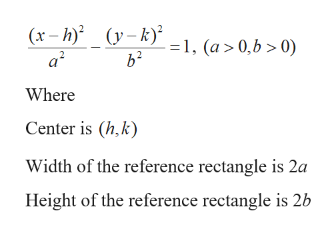# For Exercise, write the standard form of the equation of the hyperbola subject to the given conditions.Corners of the reference rectangle: (8, 7), (−6, 7), (8, −3), (−6, −3); Horizontal transverse axis

Question
33 views

For Exercise, write the standard form of the equation of the hyperbola subject to the given conditions.

Corners of the reference rectangle: (8, 7), (−6, 7), (8, −3), (−6, −3); Horizontal transverse axis

check_circle

Step 1

Given: Corners of the reference rectangle: (8, 7), (−6, 7), (8, −3), (−6, −3); Horizontal transverse axis

Step 2

The standard form of hyperbola with horizontal transverse axis is given byhelp_outlineImage Transcriptionclose(y-k)² -=1, (a > 0,b > 0) (x – h) a² Where Center is (h,k) Width of the reference rectangle is 2a Height of the reference rectangle is 2b fullscreen
Step 3

Plot the points (8, 7), (−6, 7), (8...

### Want to see the full answer?

See Solution

#### Want to see this answer and more?

Solutions are written by subject experts who are available 24/7. Questions are typically answered within 1 hour.*

See Solution
*Response times may vary by subject and question.
Tagged in

### Algebra